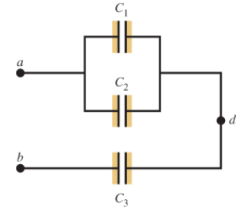# Problem: In the figure are shown three capacitors with capacitances C1 = 6.00 μF, C2 = 3.00 μF, C3 = 5.00 μF. The capacitor network is connected to an applied potential Vab. After the charges on the capacitors have reached their final values, the charge Q2 on the second capacitor is 40.0 μC. A. What is the charge Q1 on capacitor C1?B. What is the charge on capacitor C3? C. What is the applied voltage, Vab?

###### FREE Expert Solution

For capacitors in parallel, the equivalent capacitance is:

$\overline{){{\mathbf{C}}}_{\mathbf{e}\mathbf{q}}{\mathbf{=}}{{\mathbf{C}}}_{{\mathbf{1}}}{\mathbf{+}}{{\mathbf{C}}}_{{\mathbf{2}}}{\mathbf{+}}{\mathbf{.}}{\mathbf{.}}{\mathbf{.}}{\mathbf{+}}{{\mathbf{C}}}_{{\mathbf{n}}}}$

For 2 capacitors in series, the equivalent capacitance is:

$\overline{){{\mathbf{C}}}_{\mathbf{e}\mathbf{q}}{\mathbf{=}}\frac{{\mathbf{C}}_{\mathbf{1}}{\mathbf{C}}_{\mathbf{2}}}{{\mathbf{C}}_{\mathbf{1}}\mathbf{+}{\mathbf{C}}_{\mathbf{2}}}}$

The charge stored in a capacitor:

$\overline{){\mathbf{Q}}{\mathbf{=}}{\mathbf{C}}{\mathbf{V}}}$

C1 and C1 are in parallel.

Their resultant capacitance is:

C12 = 6.00 + 3.00 = 9.00 μF###### Problem Details

In the figure are shown three capacitors with capacitances C1 = 6.00 μF, C2 = 3.00 μF, C3 = 5.00 μF. The capacitor network is connected to an applied potential Vab. After the charges on the capacitors have reached their final values, the charge Q2 on the second capacitor is 40.0 μC.A. What is the charge Q1 on capacitor C1?

B. What is the charge on capacitor C3

C. What is the applied voltage, Vab?